Updating search results...

# 69 Results

View
Selected filters:
• ODE LearningOnly Sharing Permitted
CC BY-NC-ND
Rating
4.5 stars

This lesson unit is intended to help teachers assess how well students are able to visualize two-dimensional cross-sections of representations of three-dimensional objects. In particular, the lesson will help you identify and help students who have difficulties recognizing and drawing two-dimensional cross-sections at different points along a plane of a representation of a three-dimensional object.

Subject:
Geometry
Material Type:
Assessment
Lesson Plan
Provider:
Shell Center for Mathematical Education
Provider Set:
Mathematics Assessment Project (MAP)
Date Added:
04/26/2013Only Sharing Permitted
CC BY-NC-ND
Rating
0.0 stars

This lesson unit is intended to help teachers assess how well students are able to: work with concepts of congruency and similarity, including identifying corresponding sides and corresponding angles within and between triangles; Identify and understand the significance of a counter-example; Prove, and evaluate proofs in a geometric context.

Subject:
Geometry
Material Type:
Assessment
Lesson Plan
Provider:
Shell Center for Mathematical Education
Provider Set:
Mathematics Assessment Project (MAP)
Date Added:
04/26/2013Only Sharing Permitted
CC BY-NC-ND
Rating
4.0 stars

This lesson unit is intended to help you assess how well students are able to use geometric properties to solve problems. In particular, it will support you in identifying and helping students who have the following difficulties: Solving problems relating to using the measures of the interior angles of polygons; and solving problems relating to using the measures of the exterior angles of polygons.

Subject:
Geometry
Material Type:
Assessment
Lesson Plan
Provider:
Shell Center for Mathematical Education
Provider Set:
Mathematics Assessment Project (MAP)
Date Added:
04/26/2013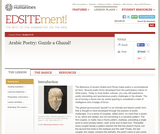Unrestricted Use
CC BY
Rating
0.0 stars

The Bedouins of ancient Arabia and Persia made poetry a conversational art form. Several poetic forms developed from the participatory nature of tribal poetry. Today in most Arabic cultures, you may still experience public storytelling and spontaneous poetry challenges in the streets. The art of turning a rhyme into sly verbal sparring is considered a mark of intelligence and a badge of honor. Students will learn about the origins and structure of Arabic Poetry.

Subject:
Arts and Humanities
Literature
World Cultures
Material Type:
Activity/Lab
Lesson Plan
Reading
Provider:
National Endowment for the Humanities
Provider Set:
EDSITEment!
Author:
Individual Authors
Date Added:
12/05/2011Read the Fine Print
Some Rights Reserved
Rating
0.0 stars

Remember your multiplication tables? ... me neither. Brush up on your multiplication, division, and factoring skills with this exciting game. No calculators allowed!

Subject:
Mathematics
Material Type:
Simulation
Provider:
University of Colorado Boulder
Provider Set:
PhET Interactive Simulations
Author:
Michael Dubson
Trish Loeblein
Date Added:
05/02/2010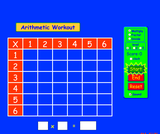Unrestricted Use
CC BY
Rating
5.0 stars

Brush up on your multiplication, division, and factoring skills with this interactive multiplication chart. Three levels and timed or untimed options are available.

Subject:
Mathematics
Material Type:
Simulation
Provider:
University of Colorado Boulder
Provider Set:
PhET Interactive Simulations
Author:
Michael Dubson
Date Added:
11/05/2011Only Sharing Permitted
CC BY-NC-ND
Rating
3.0 stars

This lesson unit is intended to help teachers assess how well students are able to create and solve linear equations. In particular, the lesson will help you identify and help students who have the following difficulties: solving equations with one variable and solving linear equations in more than one way.

Subject:
Algebra
Material Type:
Assessment
Lesson Plan
Provider:
Shell Center for Mathematical Education
Provider Set:
Mathematics Assessment Project (MAP)
Date Added:
04/26/2013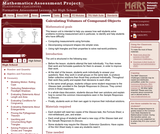Only Sharing Permitted
CC BY-NC-ND
Rating
0.0 stars

This lesson unit is intended to help teahcers assess how well students solve problems involving measurement, and in particular, to identify and help students who have the following difficulties; computing measurements using formulas; decomposing compound shapes into simpler ones; using right triangles and their properties to solve real-world problems.

Subject:
Geometry
Material Type:
Assessment
Lesson Plan
Provider:
Shell Center for Mathematical Education
Provider Set:
Mathematics Assessment Project (MAP)
Date Added:
04/26/2013Only Sharing Permitted
CC BY-NC-ND
Rating
4.0 stars

This lesson unit is intended to help teachers assess how well students are able to classify solutions to a pair of linear equations by considering their graphical representations. In particular, this unit aims to help teachers identify and assist students who have difficulties in: using substitution to complete a table of values for a linear equation; identifying a linear equation from a given table of values; and graphing and solving linear equations.

Subject:
Algebra
Material Type:
Assessment
Lesson Plan
Provider:
Shell Center for Mathematical Education
Provider Set:
Mathematics Assessment Project (MAP)
Date Added:
04/26/2013Only Sharing Permitted
CC BY-NC-ND
Rating
0.0 stars

This lesson unit is intended to help teachers assess how well students are able to interpret exponential and linear functions and in particular to identify and help students who have the following difficulties: translating between descriptive, algebraic and tabular data, and graphical representation of the functions; recognizing how, and why, a quantity changes per unit intervale; and to achieve these goals students work on simple and compound interest problems.

Subject:
Algebra
Measurement and Data
Material Type:
Assessment
Lesson Plan
Provider:
Shell Center for Mathematical Education
Provider Set:
Mathematics Assessment Project (MAP)
Date Added:
04/26/2013Only Sharing Permitted
CC BY-NC-ND
Rating
0.0 stars

This lesson unit is intended to help teachers assess how well students are able to use linear inequalities to create a set of solutions. In particular, the lesson will help teachers identify and assist students who have difficulties in: representing a constraint by shading the correct side of the inequality line; and understanding how combining inequalities affects a solution space.

Subject:
Mathematics
Material Type:
Assessment
Lesson Plan
Provider:
Shell Center for Mathematical Education
Provider Set:
Mathematics Assessment Project (MAP)
Date Added:
04/26/2013Only Sharing Permitted
CC BY-NC-ND
Rating
4.0 stars

This lesson unit is intended to help teachers assess how well students are able to: Select appropriate mathematical methods to use for an unstructured problem; interpret a problem situation, identifying constraints and variables, and specify assumptions; work with 2- and 3-dimensional shapes to solve a problem involving capacity and surface area; and communicate their reasoning clearly.

Subject:
Geometry
Material Type:
Assessment
Lesson Plan
Provider:
Shell Center for Mathematical Education
Provider Set:
Mathematics Assessment Project (MAP)
Date Added:
04/26/2013Only Sharing Permitted
CC BY-NC-ND
Rating
5.0 stars

This lesson unit is intended to help you assess whether students recognize relationships of direct proportion and how well they solve problems that involve proportional reasoning. In particular, it is intended to help you identify those students who: use inappropriate additive strategies in scaling problems, which have a multiplicative structure; rely on piecemeal and inefficient strategies such as doubling, halving, and decomposition, and have not developed a single multiplier strategy for solving proportionality problems; and see multiplication as making numbers bigger, and division as making numbers smaller.

Subject:
Numbers and Operations
Material Type:
Assessment
Lesson Plan
Provider:
Shell Center for Mathematical Education
Provider Set:
Mathematics Assessment Project (MAP)
Date Added:
04/26/2013Only Sharing Permitted
CC BY-NC-ND
Rating
0.0 stars

This lesson unit is intended to help teachers assess how well students understand the notion of correlation. In particular this unit aims to identify and help students who have difficulty in: understanding correlation as the degree of fit between two variables; making a mathematical model of a situation; testing and improving the model; communicating their reasoning clearly; and evaluating alternative models of the situation.

Subject:
Mathematics
Material Type:
Assessment
Lesson Plan
Provider:
Shell Center for Mathematical Education
Provider Set:
Mathematics Assessment Project (MAP)
Date Added:
04/26/2013Only Sharing Permitted
CC BY-NC-ND
Rating
4.5 stars

This lesson unit is intended to help assess how well students are able to interpret and use scale drawings to plan a garden layout. This involves using proportional reasoning and metric units.

Subject:
Algebra
Geometry
Ratios and Proportions
Material Type:
Assessment
Lesson Plan
Provider:
Shell Center for Mathematical Education
Provider Set:
Mathematics Assessment Project (MAP)
Date Added:
04/26/2013Only Sharing Permitted
CC BY-NC-ND
Rating
3.0 stars

This lesson unit is intended to help teachers assess how well students are able to: use the Pythagorean theorem to derive the equation of a circle; and translate between the geometric features of circles and their equations.

Subject:
Geometry
Material Type:
Assessment
Lesson Plan
Provider:
Shell Center for Mathematical Education
Provider Set:
Mathematics Assessment Project (MAP)
Date Added:
04/26/2013Only Sharing Permitted
CC BY-NC-ND
Rating
0.0 stars

This lesson unit is intended to help teachers assess how well students are able to: translate between the equations of circles and their geometric features; and sketch a circle from its equation.

Subject:
Geometry
Material Type:
Assessment
Lesson Plan
Provider:
Shell Center for Mathematical Education
Provider Set:
Mathematics Assessment Project (MAP)
Date Added:
04/26/2013Only Sharing Permitted
CC BY-NC-ND
Rating
4.0 stars

This lesson unit is intended to help you assess how well students are able to: solve simple problems involving ratio and direct proportion; choose an appropriate sampling method; and collect discrete data and record them using a frequency table.

Subject:
Education
Geometry
Measurement and Data
Ratios and Proportions
Material Type:
Assessment
Lecture Notes
Lesson Plan
Teaching/Learning Strategy
Provider:
Shell Center for Mathematical Education
Provider Set:
Mathematics Assessment Project (MAP)
Author:
http://map.mathshell.org/
Date Added:
04/26/2013Only Sharing Permitted
CC BY-NC-ND
Rating
4,0 stars

This lesson unit is intended to help teachers assess how well students are able to: estimate lengths of everyday objects; convert between decimal and scientific notation; and make comparisons of the size of numbers expressed in both decimal and scientific notation.

الموضوع:
الرياضيات
نوع المادة:
التقييم
Lesson Plan
Provider:
Shell Center for Mathematical Education
Provider Set:
Mathematics Assessment Project (MAP)
Date Added:
04/26/2013Only Sharing Permitted
CC BY-NC-ND
Rating
4.0 stars

This lesson unit is intended to help you assess how well students are able to: Model a situation; make sensible, realistic assumptions and estimates; and use assumptions and estimates to create a chain of reasoning, in order to solve a practical problem.

Subject:
Geometry
Material Type:
Assessment
Lesson Plan
Provider:
Shell Center for Mathematical Education
Provider Set:
Mathematics Assessment Project (MAP)
Date Added:
04/26/2013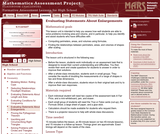Only Sharing Permitted
CC BY-NC-ND
Rating
0.0 stars

This lesson unit is intended to help teachers assess how well students are able to solve problems involving area and volume, and in particular, to help you identify and assist students who have difficulties with the following: computing perimeters, areas and volumes using formulas; and finding the relationships between perimeters, areas, and volumes of shapes after scaling.

Subject:
Geometry
Material Type:
Assessment
Lesson Plan
Provider:
Shell Center for Mathematical Education
Provider Set:
Mathematics Assessment Project (MAP)
Date Added:
04/26/2013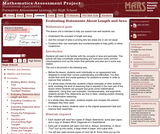Only Sharing Permitted
CC BY-NC-ND
Rating
0.0 stars

This lesson unit is intended to help teachers assess how well students can: Understand the concepts of length and area; use the concept of area in proving why two areas are or are not equal; and construct their own examples and counterexamples to help justify or refute conjectures.

Subject:
Geometry
Material Type:
Assessment
Lesson Plan
Provider:
Shell Center for Mathematical Education
Provider Set:
Mathematics Assessment Project (MAP)
Date Added:
04/26/2013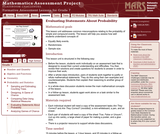Only Sharing Permitted
CC BY-NC-ND
Rating
4,0 stars

This lesson unit addresses common misconceptions relating to probability of simple and compound events. The lesson will help you assess how well students understand concepts of: Equally likely events; randomness; and sample sizes.

الموضوع:
الإحصائيات والاحتمالات
نوع المادة:
التقييم
Lesson Plan
Provider:
Shell Center for Mathematical Education
Provider Set:
Mathematics Assessment Project (MAP)
Date Added:
04/26/2013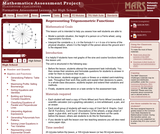Only Sharing Permitted
CC BY-NC-ND
Rating
0.0 stars

This lesson unit is intended to help teachers assess how well students are able to: model a periodic situation, the height of a person on a Ferris wheel, using trigonometric functions; and interpret the constants a, b, c in the formula h = a + b cos ct in terms of the physical situation, where h is the height of the person above the ground and t is the elapsed time.

Subject:
Functions
Material Type:
Assessment
Lesson Plan
Provider:
Shell Center for Mathematical Education
Provider Set:
Mathematics Assessment Project (MAP)
Date Added:
04/26/2013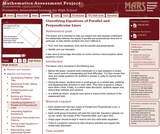Only Sharing Permitted
CC BY-NC-ND
Rating
0.0 stars

This lesson unit is intended to help teachers assess how well students are able to understand the relationship between the slopes of parallel and perpendicular lines and, in particular, to help identify students who find it difficult to: find, from their equations, lines that are parallel and perpendicular; and identify and use intercepts. It also aims to encourage discussion on some common misconceptions about equations of lines.

Subject:
Mathematics
Material Type:
Assessment
Lesson Plan
Provider:
Shell Center for Mathematical Education
Provider Set:
Mathematics Assessment Project (MAP)
Date Added:
04/26/2013Only Sharing Permitted
CC BY-NC-ND
Rating
0.0 stars

This lesson unit is intended to help teachers assess how well students are able to understand what the different algebraic forms of a quadratic function reveal about the properties of its graphical representation. In particular, the lesson will help teachers identify and help students who have the following difficulties: understanding how the factored form of the function can identify a graphŐs roots; understanding how the completed square form of the function can identify a graphŐs maximum or minimum point; and understanding how the standard form of the function can identify a graphŐs intercept.

Subject:
Algebra
Material Type:
Assessment
Lesson Plan
Provider:
Shell Center for Mathematical Education
Provider Set:
Mathematics Assessment Project (MAP)
Date Added:
04/26/2013Only Sharing Permitted
CC BY-NC-ND
Rating
0.0 stars

This lesson unit is intended to help teachers assess how well students are able to: articulate verbally the relationships between variables arising in everyday contexts; translate between everyday situations and sketch graphs of relationships between variables; interpret algebraic functions in terms of the contexts in which they arise; and reflect on the domains of everyday functions and in particular whether they should be discrete or continuous.

Subject:
Functions
Material Type:
Assessment
Lesson Plan
Provider:
Shell Center for Mathematical Education
Provider Set:
Mathematics Assessment Project (MAP)
Date Added:
04/26/2013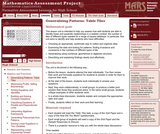Only Sharing Permitted
CC BY-NC-ND
Rating
0.0 stars

This lesson unit is intended to help teachers assess how well students are able to identify linear and quadratic relationships in a realistic context: the number of tiles of different types that are needed for a range of square tabletops. In particular, this unit aims to identify and help students who have difficulties with: choosing an appropriate, systematic way to collect and organize data; examining the data and looking for patterns; finding invariance and covariance in the numbers of different types of tile; generalizing using numerical, geometrical or algebraic structure; and describing and explaining findings clearly and effectively.

Subject:
Algebra
Geometry
Measurement and Data
Material Type:
Assessment
Lesson Plan
Provider:
Shell Center for Mathematical Education
Provider Set:
Mathematics Assessment Project (MAP)
Date Added:
04/26/2013Only Sharing Permitted
CC BY-NC-ND
Rating
3,0 stars

This lesson unit is intended to help you assess how well students working with square numbers are able to: choose an appropriate, systematic way to collect and organize data, examining the data for patterns; describe and explain findings clearly and effectively; generalize using numerical, geometrical, graphical and/or algebraic structure; and explain why certain results are possible/impossible, moving towards a proof.

الموضوع:
علم الجبر
هندسة الشكل
القياس والبيانات
نوع المادة:
التقييم
Lesson Plan
Provider:
Shell Center for Mathematical Education
Provider Set:
Mathematics Assessment Project (MAP)
Date Added:
04/26/2013Only Sharing Permitted
CC BY-NC-ND
Rating
0.0 stars

This lesson unit is intended to help teachers assess how well students are able to use geometric properties to solve problems. In particular, the lesson will help you identify and help students who have the following difficulties: solving problems by determining the lengths of the sides in right triangles; and finding the measurements of shapes by decomposing complex shapes into simpler ones. The lesson unit will also help students to recognize that there may be different approaches to geometrical problems, and to understand the relative strengths and weaknesses of those approaches.

Subject:
Geometry
Material Type:
Assessment
Lesson Plan
Provider:
Shell Center for Mathematical Education
Provider Set:
Mathematics Assessment Project (MAP)
Date Added:
04/26/2013Only Sharing Permitted
CC BY-NC-ND
Rating
4.0 stars

This lesson unit is intended to help you assess how students reason about geometry and, in particular, how well they are able to: use facts about the angle sum and exterior angles of triangles to calculate missing angles; apply angle theorems to parallel lines cut by a transversal; interpret geometrical diagrams using mathematical properties to identify similarity of triangles.

Subject:
Geometry
Material Type:
Assessment
Lesson Plan
Provider:
Shell Center for Mathematical Education
Provider Set:
Mathematics Assessment Project (MAP)
Date Added:
04/26/2013Only Sharing Permitted
CC BY-NC-ND
Rating
4.0 stars

This lesson unit is intended to help teachers assess how well students are able to interpret percent increase and decrease, and in particular, to identify and help students who have the following difficulties: translating between percents, decimals, and fractions; representing percent increase and decrease as multiplication; and recognizing the relationship between increases and decreases.

Subject:
Ratios and Proportions
Material Type:
Assessment
Lesson Plan
Provider:
Shell Center for Mathematical Education
Provider Set:
Mathematics Assessment Project (MAP)
Date Added:
04/26/2013Only Sharing Permitted
CC BY-NC-ND
Rating
0.0 stars

This lesson unit is intended to help teachers assess how well students are able to use geometric properties to solve problems. In particular, it will help you identify and help students who have difficulty: decomposing complex shapes into simpler ones in order to solve a problem; bringing together several geometric concepts to solve a problem; and finding the relationship between radii of inscribed and circumscribed circles of right triangles.

Subject:
Geometry
Material Type:
Assessment
Lesson Plan
Provider:
Shell Center for Mathematical Education
Provider Set:
Mathematics Assessment Project (MAP)
Date Added:
04/26/2013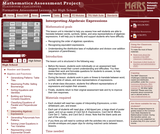Only Sharing Permitted
CC BY-NC-ND
Rating
0.0 stars

This lesson unit is intended to help teachers assess how well students are able to translate between words, symbols, tables, and area representations of algebraic expressions. It will help teachers to identify and support students who have difficulty in: recognizing the order of algebraic operations; recognizing equivalent expressions; and understanding the distributive laws of multiplication and division over addition (expansion of parentheses).

Subject:
Algebra
Material Type:
Assessment
Lesson Plan
Provider:
Shell Center for Mathematical Education
Provider Set:
Mathematics Assessment Project (MAP)
Date Added:
04/26/2013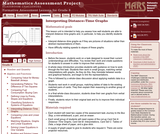Only Sharing Permitted
CC BY-NC-ND
Rating
4.0 stars

This lesson unit is intended to help teachers assess how well students are able to interpret distanceĐtime graphs and, in particular, to help you identify students who: interpret distanceĐtime graphs as if they are pictures of situations rather than abstract representations of them; and have difficulty relating speeds to slopes of these graphs.

Subject:
Mathematics
Material Type:
Assessment
Lesson Plan
Provider:
Shell Center for Mathematical Education
Provider Set:
Mathematics Assessment Project (MAP)
Date Added:
04/26/2013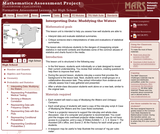Only Sharing Permitted
CC BY-NC-ND
Rating
0,0 stars

This lesson unit is intended to help teachers assess how well students are able to: interpret data and evaluate statistical summaries; and critique someone elseŐs interpretations of data and evaluations of statistical summaries. The lesson also introduces students to the dangers of misapplying simple statistics in real-world contexts, and illustrates some of the common abuses of statistics and charts found in the media.

الموضوع:
الإحصائيات والاحتمالات
نوع المادة:
التقييم
Lesson Plan
Provider:
Shell Center for Mathematical Education
Provider Set:
Mathematics Assessment Project (MAP)
Date Added:
04/26/2013Only Sharing Permitted
CC BY-NC-ND
Rating
4.0 stars

This lesson unit is intended to help you assess how well students are able to: Perform arithmetic operations, including those involving whole-number exponents, recognizing and applying the conventional order of operations; Write and evaluate numerical expressions from diagrammatic representations and be able to identify equivalent expressions; apply the distributive and commutative properties appropriately; and use the method for finding areas of compound rectangles.

Subject:
Geometry
Material Type:
Assessment
Lesson Plan
Provider:
Shell Center for Mathematical Education
Provider Set:
Mathematics Assessment Project (MAP)
Date Added:
04/26/2013Only Sharing Permitted
CC BY-NC-ND
Rating
5,0 stars

This lesson unit is intended to help teahcers assess how well students are able to interpret speed as the slope of a linear graph and translate between the equation of a line and its graphical representation.

الموضوع:
علم الجبر
نوع المادة:
التقييم
Lesson Plan
Provider:
Shell Center for Mathematical Education
Provider Set:
Mathematics Assessment Project (MAP)
Date Added:
04/26/2013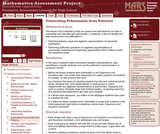Only Sharing Permitted
CC BY-NC-ND
Rating
0.0 stars

This lesson unit is intended to help you assess how well students are able to manipulate and calculate with polynomials. In particular, it aims to identify and help students who have difficulties in: switching between visual and algebraic representations of polynomial expressions; and performing arithmetic operations on algebraic representations of polynomials, factorizing and expanding appropriately when it helps to make the operations easier.

Subject:
Mathematics
Material Type:
Assessment
Lesson Plan
Provider:
Shell Center for Mathematical Education
Provider Set:
Mathematics Assessment Project (MAP)
Date Added:
04/26/2013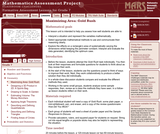Only Sharing Permitted
CC BY-NC-ND
Rating
3.0 stars

This lesson unit is intended to help you assess how well students are able to: Interpret a situation and represent the variables mathematically; select appropriate mathematical methods to use; explore the effects on the area of a rectangle of systematically varying the dimensions whilst keeping the perimeter constant; interpret and evaluate the data generated and identify the optimum case; and communicate their reasoning clearly.

Subject:
Geometry
Material Type:
Assessment
Lesson Plan
Provider:
Shell Center for Mathematical Education
Provider Set:
Mathematics Assessment Project (MAP)
Date Added:
04/26/2013